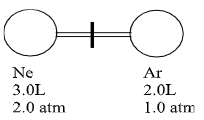# Problem: In the flask diagrammed below, the left bulb contains 3.0L of neon at a pressure of 2.0 atm. The right bulb contains 2.0L of argon at a pressure of 1.0 atm. What is the mole fraction of neon and argon in the final mixture after the valve is opened and a uniform pressure is reached at constant temperature (25°C).(a) Ne 0.33, Ar 0.67(b) Ne 0.25, Ar 0.75(c) Ne 0.67, Ar 0.33(d) Ne 0.92, Ar 0.82(e) Ne 0.75, Ar 0.25

###### FREE Expert Solution

We are asked to calculate the mole fractions of each of the gases.

Mole Fraction (X) relates the moles of components and the total moles:

Now to get the total moles we have:

So for the mole fraction of Ne and Ar we have:

83% (329 ratings)###### Problem Details

In the flask diagrammed below, the left bulb contains 3.0L of neon at a pressure of 2.0 atm. The right bulb contains 2.0L of argon at a pressure of 1.0 atm. What is the mole fraction of neon and argon in the final mixture after the valve is opened and a uniform pressure is reached at constant temperature (25°C).(a) Ne 0.33, Ar 0.67

(b) Ne 0.25, Ar 0.75

(c) Ne 0.67, Ar 0.33

(d) Ne 0.92, Ar 0.82

(e) Ne 0.75, Ar 0.25